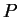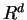Next: What computer models are Up: Convex Polyhedron Previous: What is the vertex   Contents

## How can one enumerate all faces of a convex polyhedron?

Letbe a convex polytope in. One can extend the discussion below for the unbounded case (polyhedron) by adding a face at infinity, but for simplicity we assumeis bounded.

First of all the answer does not depend on howis given. The problem for H-polytopes is equivalent to the one for V-polytopes by duality. See Sections 2.11 and 2.4.

There are algorithms (e.g. [Rot92,Sei86,FLM97] ) that can generate all faces from a V-representation or from a H-rerepsentation. Perhaps the backtrack algorithm [FLM97] is easiest to implement and works directly for the unbounded case. It is also a compact polynomial algorithm (see 2.15) and thus needs little space to run. Algorithms that need to store all faces during computation tend to be too complicated to implement, because one needs to manage a complex data structure of faces and their incidences.

Another approach to generate all faces consists of two steps.

(1)
Firstly compute the second representation by a representation conversion algorithm.
(2)
Secondly use a combinatorial method to genrate all faces.
The first part is discussed in Section 2.15 and Section 5 presents some existing implementation. The second part can be done efficiently by purely combinatorial computation, see [FR94]. As explained in [FR94], when the polytope is simple (simplicial), the face listing without duplication can be done implicitely by sorting the vertices (the facets) by a generic linear function (a generic line through an interior point).Next: What computer models are Up: Convex Polyhedron Previous: What is the vertex   Contents
Komei Fukuda 2004-08-26